## Wednesday, August 24, 2016

### Back to basics: a very simple scheduling problem

In this post an extremely simple scheduling problem is proposed. But as we shall see, there are still a few interesting things to say about this. There are $$n=9$$ persons, two of which need to be available on-call each weekend. We need to design a schedule such that:

1. If a pair $$(i,j)$$ covers a weekend, then this same pair can not cover another weekend during the planning horizon. Persons $$i$$ and $$j$$ can form other pairs and in that configuration cover other weekends.
2. If a person $$i$$ covers a weekend $$t$$, he/she is off the next two weekends.

With $$n=9$$ persons we have $$N=36$$ possible pairs. The number is the same as the number of strictly sub-diagonal elements in a $$n \times n$$ matrix. This can be derived as $$N=(n-1)+(n-2)+…+1+0 = \frac{1}{2}n(n-1)$$. The number $$N=36$$ also dictates our planning window: if we have more than 36 weeks we need to recycle pairs.

##### Variables and equations

I propose to model this with two sets of binary variables:

• $$x_{i,t} \in \{0,1\}$$ is the assignment of person $$i$$ to duty on weekend $$t$$.
• $$y_{i,j,t}\in \{0,1\}$$ is the assignment of pair $$(i,j)$$ to weekend $$t$$. We say $$(i,j)\in P$$ to indicate we consider these unique $$N=36$$ pairs and not the full Carthesian product.

The rather obvious constraints on variable $$y$$ are:

 \begin{align} & \sum_{(i,j)\in P} y_{i,j,t} = 1 & \forall t & \>\>\> \text{(1)} \\ & \sum_{t} y_{i,j,t} \le 1 & \forall (i,j) \in P & \>\>\>\text{(2)} \end{align}
Here equation (1) indicates we want exactly one pair per weekend and equation (2) implements no repeating occurrences of a pair $$(i,j)$$.

The constraint on variable $$x$$ is the implication: $$x_{i,t}=1 \implies x_{i,t+1}=x_{i,t+2}=0$$. Interestingly we can simply implement this as:

 $x_{i,t}+x_{i,t+1}+x_{i,t+2}\le 1\>\>\>\forall i,t\>\>\> \text{(3)}$

The equivalence of these two restrictions may need a little bit of thought. It will prevent sequences like $$[1,1]$$ and $$[1,0,1]$$. We may need to pay a little bit of attention near the end of the planning period. In GAMS leads and lags that exceed the domain are automatically zero, and that is actually the correct behavior here. We end up with a last equation $$x_{i,T}+0+0\le 1$$ which we will assume the pre-solver of the MIP solver will remove. In AMPL you will need to handle the border conditions explicitly.

It is noted that equation (1) can also be written in terms of $$x$$. I.e. we could replace (1) by:

 $\sum_i x_{i,t} = 2\>\>\>\forall t \>\>\> \text{(1a)}$

Finally we need connect $$x$$ and $$y$$. We can do this by $$y_{i,j,t}=x_{i,t}\cdot x_{j,t}$$. However this is non-linear. We can linearize this binary multiplication by:

 \boxed{\begin{align}&y_{i,j,t}\le x_{i,t}\\ &y_{i,j,t}\le x_{j,t}\\&y_{i,j,t}\ge x_{i,t}+x_{j,t}-1 \end{align}} $$\forall (i,j)\in P, t$$ $$\>\>\text{(4)}$$

If we use equation (1a) instead of (1) we can actually drop the first two inequalities of equation (4). This requires again a bit of thought. This step leaves $$y$$ floating in some cases. In essence we have relaxed the definition of $$y$$ somewhat: we use now $$y_{i,j,t}\ge x_{i,t}\cdot x_{j,t}$$. So when using this formulation, we should make sure to use only $$x$$ when reporting results. As noted in the comments, actually when using $$T=36$$, $$y$$ forms a proper schedule (Q: explain why) but when $$T<36$$ these floating $$y$$’s can be misleading as there may be spurious $$y$$’s being turned on. Hence, in this case only look at $$x$$ when reporting solutions. Of course if we want we can recover $$y$$ after the solve by evaluating $$y^*_{i,j,t} := x^*_{i,t}\cdot x^*_{j,t}$$.

There is no objective. As there is no objective the solver will stop at the first integer feasible point. This also means we do not need to worry about setting a gap tolerance optcr: the first integer feasible point found is immediately proven optimal. A constraint programming (CP) solver typically allow for a simpler representation of scheduling models, and I am pretty sure this will also be the case for this model.

##### Complete model and results

The complete model can look like: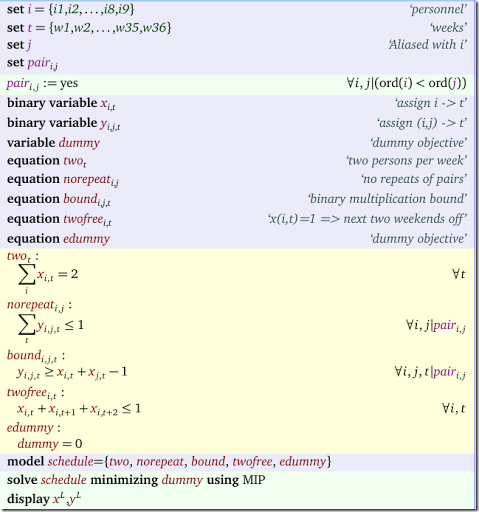A solution can look like: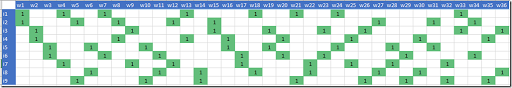##### Infinite horizon

In the model above we used a planning horizon of $$N=36$$ weeks. One way of dealing with longer horizons is to recycle the schedule: apply the schedule for week 1 to week 37. In this case we would have a violation of the two-weeks off rule: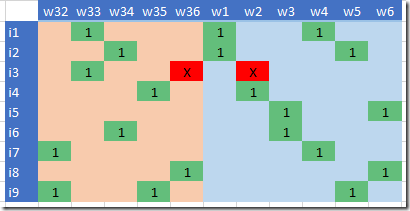Fixing this is not too complicated. We need to change equation (3) as follows:

 \boxed{\begin{align}&x_{i,t}+x_{i,t+1}+x_{i,t+2}\le 1\>\>\>&\forall i,t\>\>&\forall i\\&x_{i,T}+x_{i,1}+x_{i,2}\>\>\>&\forall i \end{align}} (3a)

where $$T=36$$ indicates the last week of the planning period.

In GAMS we can use the ++ operator for this and we can just write simply: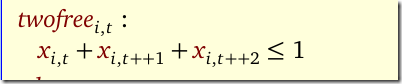With this change the resulting schedule is: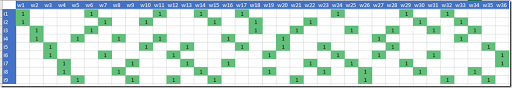Now we have a proper periodic schedule and we can recycle it starting in week 37. With this, we effectively have designed a steady state schedule that can be repeated ad infinitum. In other words, we have created a schedule with an infinite planning horizon.